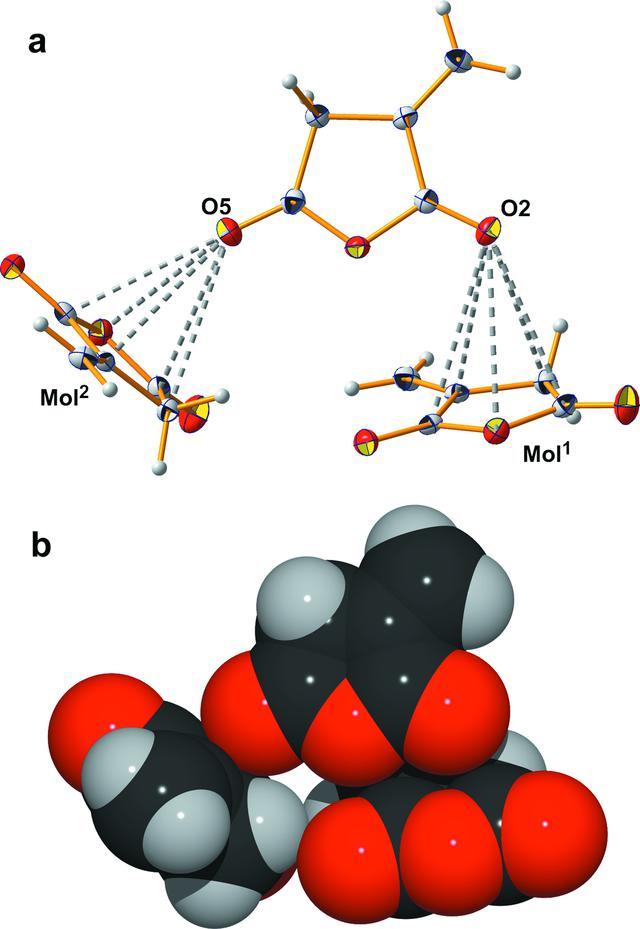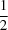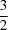disable zoom     view article Figure 5 Ball-and-stick model (a) and space-filling model (b) of the inter­action (dashed stick, grey) of the carbonyl oxygen atoms with the ring atoms of neighbouring mol­ecules; with exception of the hydrogen atoms, which are shown as spheres with common isotropic radius, all other atoms are represented as thermal displacement ellipsoids showing the 50% probability level of the corresponding atom; distances: d(O5⋯O12) = 3.097 (2) Å, d(O5⋯C22) = 3.083 (2) Å, d(O5⋯C32) = 3.131 (2) Å, d(O5⋯C42) = 3.173 (2) Å, d(O5⋯C52) = 3.165 (2) Å; d(O2⋯O11) = 3.389 (2) Å, d(O2⋯C51) = 3.210 (2) Å, d(O2⋯C41) = 3.181 (2) Å, d(O2⋯C31) = 3.277 (2) Å, d(O2⋯ C21) = 3.419 (2) Å. [Symmetry codes: (1)+ x,− y, 1 − z; (2) 2 − x, −+ y,− z]CRYSTALLOGRAPHICCOMMUNICATIONS
ISSN: 2056-9890